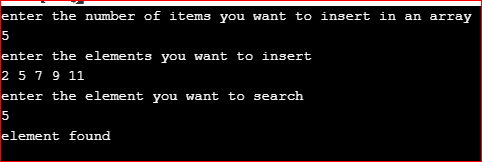# Foreach Loop in C++

## Foreach Loop in C++ with Examples:

In this article, I am going to discuss Foreach Loop in C++ Language with Examples. Please read our previous articles, where we discussed Arrays in C++ with Examples.

##### Foreach Loop in C++:

This loop is used with a collection of elements, so a collection of elements is nothing but an array. for each loop works with the array. This is the feature of C++11. So already we have learned about array. We have shown you that the ‘for’ loop is used for accessing all elements of an array.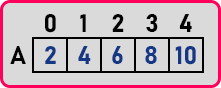Array name is ‘A’ and there are five elements. Now for displaying all the elements I can use ‘for’ loop that will iterate through all these elements or scan through all these elements. So for loop is a counter control loop.

for(int i = 0; i < 5; i++){
cout << A[i] << endl;
}

So how does it work?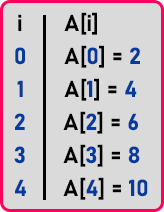Initially ‘i’ is 0, here A[i] means A which is ‘2’. So ‘2’ will print and ‘i’ will be incremented. Now ‘i’ is 1, here A[i] means A which is ‘4’. So ‘4’ will print and ‘i’ will be incremented and so on till we reach the end of the array. In this loop, ‘i’ is taking the indices of elements. Now the same thing we can access using ‘for each’ loop. Let us see this syntax and example,

for(int x: A)
cout << x << endl;
}

The line ‘int x: A’ means ‘x in A’. So let us see how it works. The first time, ‘x’ will be ‘2’, x is 2, so ‘x’ is a variable in which the first value of the array will be copied. So, when we print ‘x’, 2 will be printed,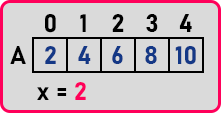Next time inside the same ‘x’. ‘4’ will be copied and printed.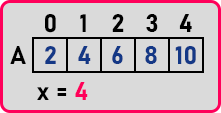In the ‘for’ loop, ‘i’ was the index but in ‘foreach’ loop ‘x’ is the element itself. So, this ‘x’ will get all the values from an array one by one. In the ‘for’ loop you have to mention the size i.e. 5 so you should stop indexing at the 4. But in the ‘foreach’ loop there is no size this is the benefit of the ‘foreach’ loop. So, this is dependent on the size. This is not dependent on the size it will access all the elements, as many elements are there. Now one more thing, if we write,

for(int x: A)
cout << x << endl;
}

Here we write ‘++x’ so the first value will be ‘2’ then it will print ‘++x’ means ‘3’. The original value of the array will not change because ‘x’ is getting a copy of that value. ‘x’ is a separate variable. Then the next time it will get ‘4’, then ‘++x’. This will become ‘5’ and ‘5’ is printed but the original value remains ‘4’ only. So, the point we have to show you is that this ‘x’ will get the copy of a value. Now if I want to modify that value, ‘x’ should represent that value directly, you should not have a copy. Then we have to add references as,

for(int &x: A)
cout << x << endl;
}

Reference ‘&x’ will give a name to the same value. That is another name for the same value. We will discuss references in a different article but for here just remember this. If we don’t give a reference then that value will not modify.

Now one more benefit of using for each loop we will show you. There is an array ‘A’, we know A is there but we don’t know its data type. What data type we should declare, int, float, or double? Instead of defining the data type by ourselves, we can simply say ‘auto’ as

for(auto x: A)
cout << x << endl;
}

so automatically the compiler will make this variable ‘x’ the same as the data type of this array. This is a more powerful feature of C++ along with the ‘foreach’ loop and one more point about the foreach loop is that it will work on a collection of elements only either it may be an array or vector or any other thing that we will study later on but it will not work on pointers right.

##### Program to print Array using Foreach Loop in C++:
```#include <iostream>
using namespace std;
int main()
{
int A = { 2, 4, 6, 8, 10 };
for(int x:A)
{
cout << x;
}
}
```
###### Output: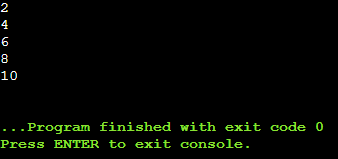##### Advantages of Using Foreach loop in C++:

The advantage of using Foreach loop on arrays is it avoids programming errors done by the developer. For Example, in a regular for loop if the programmer wrongly mentioned the size of an array, then it will lead to the problem but in a Foreach loop sizeof an array is taken implicitly and there is no need to specify the size of an array explicitly and no need to initialize the variable also.

##### Flow Diagram of Foreach Loop: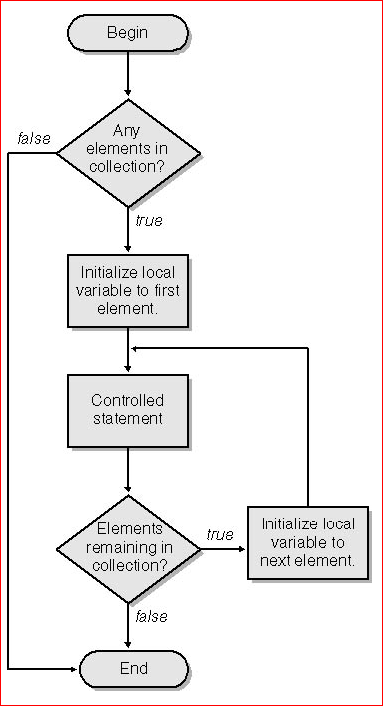Now we know how to use Foreach loop and its importance. Let’s write a program to search for an element in an array i.e. popularly called linear search.

```#include <iostream>
using namespace std;
int main()
{
int num_of_items;
cout <<"enter the number of items you want to insert in an array" << endl;
cin >> num_of_items;
int items[num_of_items];
cout <<"enter the elements you want to insert" << endl;

for(int i = 0; i < num_of_items; i++)
{
cin >> items[i];
}
int key;
cout <<"enter the element you want to search" << endl;
cin >> key;
for(int i:items)
{
if(i == key)
{
cout <<"element found" << endl;
return 0;
}
}